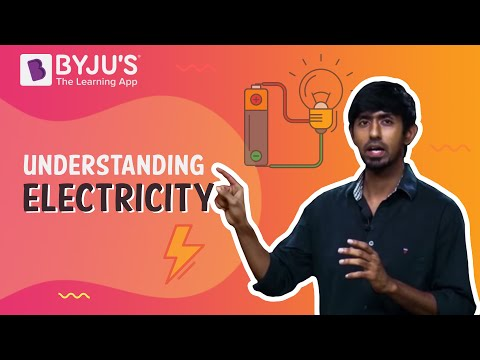# Unit of Current

## Introduction:

We have heard a lot about electric currents in our classrooms and at home. While the topic is highly relatable, in more scientific terms an electric current is basically a flow of current or charge in electric circuits.

Sometimes both ions and electrons carry the charge simultaneously. Electric current or charge is measured by an ammeter, and there are different measurement methods as well as units of current. Here we will look at some of them in detail.

For a quick revision on electricity, watch the video below.## What is the SI Unit of Electric Current?

The SI unit of current is ampere which measures the flow of electric charge across a surface at the rate of one coulomb per second. Since the charge is measured in coulombs and time in seconds, so the unit is coulomb/Sec (C/s) or amp. Meanwhile, the formula for electric current is given as follows:

I = V/R

Where,

• I = Electric Current
• V = Voltage
• R = Resistance of the material

Ampere is one of the SI base units for electric current. It is used in electronic and electrical science as well as other areas of science. The definition of ampere is based on the electromagnetic effect it induces.

You may also want to check out these topics given below!

### Some Other Current Units

Apart from ampere, there are several standard units of measurement used for the expression of electrical properties like the voltage, resistance, power, capacitance, inductance, electric field, electric charge, frequency, the magnetic flux which are all related to electric current.

 Electrical Parameter Measuring Unit Symbol Voltage Volt V or E Resistance Ohm R or Ω Capacitance Farad C Charge Coulomb Q Inductance Henry L or H Power Watts W Impedance Ohm Z Frequency Hertz Hz Conductance Siemen G or ℧

## Frequently Asked Questions – FAQs

Q1

### What is the definition of electric current?

An electric current is a flow of ions or electrons travelling through space or an electric conductor.
Q2

### What is the SI unit of current?

The SI unit of current is ampere.
Q3

### What is the formula of current?

The formula of current is:
I = V/R
Q4

### What are the basic two types of current?

The basic two types of current are alternating current (AC) and direct current (DC).
Q5

Electrons